The CANDISC Procedure

Computational Details

General Formulas

Canonical discriminant analysis is equivalent to canonical correlation analysis between the quantitative variables and a set of dummy variables coded from the class variable. In the following notation the dummy variables are denoted byand the quantitative variables by. The total sample covariance matrix for theandvariables isWhen c is the number of groups,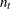is the number of observations in group t, and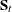is the sample covariance matrix for thevariables in group t, the within-class pooled covariance matrix for thevariables is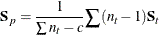The canonical correlations,, are the square roots of the eigenvalues,, of the following matrix. The corresponding eigenvectors are.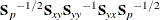Letbe the matrix with the eigenvectorsthat correspond to nonzero eigenvalues as columns. The raw canonical coefficients are calculated as follows: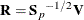The pooled within-class standardized canonical coefficients are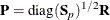The total sample standardized canonical coefficients areLet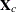be the matrix with the centeredvariables as columns. The canonical scores can be calculated by any of the following: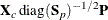For the multivariate tests based on,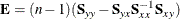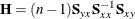where n is the total number of observations.